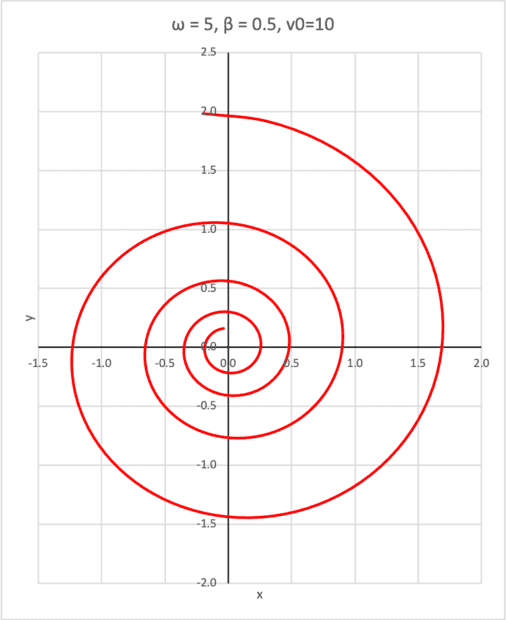# Solved problem: Particle trajectory with fixed angle between acceleration and velocity

Homework Helper
Gold Member
Homework Statement:
Find the trajectory of a particle the acceleration of which is fixed at angle ##\varphi > \pi/2## relative to the velocity.
Relevant Equations:
Newton's second law.
This is a solution to a problem inspired by another thread. It is posted here to separate it from the multiple choice question which was the subject of that thread. A parametric solution for the trajectory can be found quite easily if the motion is modeled as a particle with charge ##q## moving in a uniform magnetic field ##\mathbf{B}=B~\mathbf{\hat z}##. In addition to the Lorentz force, air resistance provides retarding force ##\mathbf{F}_{\text{ret.}}=-b\mathbf{v}.##

First we prove that this model predicts a constant angle between acceleration and velocity and find an expression for the angle ##\varphi## between acceleration and velocity. We assume that the particle moves in the ##xy##-plane. From Newton's second law we have \begin{align}\mathbf{a}=\frac{1}{m}(q\mathbf{v}\times\mathbf{B}-b\mathbf{v}).\end{align}Then, noting that ##\mathbf{v}\cdot(\mathbf{v}\times\mathbf{B})=0##, $$\mathbf{v}\cdot\mathbf{a}=\frac{1}{m}\mathbf{v}\cdot[q(\mathbf{v}\times\mathbf{B})-b\mathbf{v}]=-\frac{bv^2}{m}$$Also, \begin{align} \mathbf{a}\cdot\mathbf{a} =a^2 & =\frac{1}{m}(q\mathbf{v}\times\mathbf{B}-b\mathbf{v})\cdot \frac{1}{m}(q\mathbf{v}\times\mathbf{B}-b\mathbf{v})\nonumber \\ & =\frac{1}{m^2}({q^2B^2v^2+b^2v^2})\implies a=\frac{1}{m}(q^2 B^2 v^2+b^2 v^2)^{1/2}.\nonumber \end{align}The cosine of the angle between acceleration and velocity is constant and given by \begin{align} \cos\!\varphi=\frac{\mathbf{v}\cdot\mathbf{a}}{va}=-\frac{b}{(q^2B^2+b^2)^{1/2}}\end{align}. We now write Newton's second law in two dimensions to find the trajectory. To simplify the form of the equations we use the cyclotron frequency ##\omega_c=qB/m## and auxiliary variable ##\beta=b/m##. Then from equation (1) the components of the acceleration are
\begin{align} & \dot v_x =\omega_c v_y-\beta v_x \\ & \dot v_y=-\omega_c v_x-\beta v_y. \end{align}The two coupled equations can be solved quite easily by changing variables, $$\xi=v_x+iv_y~;~~\eta = v_x-iv_y$$ in which case equations (3) and (4) become \begin{align} & \dot{\xi}+\dot{\eta}=-i\omega_c(\xi-\eta)-\beta(\xi+\eta) \nonumber \\&\dot{\xi}-\dot{\eta}=-i\omega_c(\xi+\eta)-\beta(\xi-\eta). \nonumber \end{align} Adding the equations yields \begin{align} &\dot {\xi} =-(i\omega_c+\beta)\xi\implies \xi=Ae^{-\beta t}e^{-i\omega_c t} \nonumber \\ & \dot{\eta}=\dot {\xi}^*= A^*e^{-\beta t}e^{i\omega_c t} .\nonumber \end{align}We can now employ the definitions for ##\xi## and ##\eta## and use the initial conditions ##v_x(0)=v_0## and ##v_y(0)=0## to find \begin{align} & v_x(t)=v_0e^{-\beta t}\cos(\omega_c t) \nonumber \\ & v_y(t) = v_0e^{-\beta t}\sin(\omega_c t). \nonumber \end{align}Finally, we integrate to find ##x(t)## and ##y(t)## such that ##v_x(0)=v_0## and ##v_y(0)=0##: \begin{align} & x(t)=\frac{v_0e^{-\beta t}}{\beta^2+\omega_c^2}[\omega_c\sin(\omega_c t)-\beta \cos(\omega_c t)]\nonumber \\ & y(t)=\frac{v_0e^{-\beta t}}{\beta^2+\omega_c^2}[\omega_c\cos(\omega_c t)+\beta \sin(\omega_c t)]\nonumber \end{align} The graph below is a parametric plot of the trajectory with parameters as shown.Last edited:
•••berkeman, DrClaude, PeroK and 5 others

Gold Member
It's going to take some dissecting to see how far off I was. Thanks for sharing!

•Callumnc1 and kuruman
Callumnc1
Homework Statement:: Find the trajectory of a particle the acceleration of which is fixed at angle ##\varphi > \pi/2## relative to the velocity.
Relevant Equations:: Newton's second law.

This is a solution to a problem inspired by another thread. It is posted here to separate it from the multiple choice question which was the subject of that thread. A parametric solution for the trajectory can be found quite easily if the motion is modeled as a particle with charge ##q## moving in a uniform magnetic field ##\mathbf{B}=B~\mathbf{\hat z}##. In addition to the Lorentz force, air resistance provides retarding force ##\mathbf{F}_{\text{ret.}}=-b\mathbf{v}.##

First we prove that this model predicts a constant angle between acceleration and velocity and find an expression for the angle ##\varphi## between acceleration and velocity. We assume that the particle moves in the ##xy##-plane. From Newton's second law we have \begin{align}\mathbf{a}=\frac{1}{m}(q\mathbf{v}\times\mathbf{B}-b\mathbf{v}).\end{align}Then, noting that ##\mathbf{v}\cdot(\mathbf{v}\times\mathbf{B})=0##, $$\mathbf{v}\cdot\mathbf{a}=\frac{1}{m}\mathbf{v}\cdot[q(\mathbf{v}\times\mathbf{B})-b\mathbf{v}]=-\frac{bv^2}{m}$$Also, \begin{align} \mathbf{a}\cdot\mathbf{a} =a^2 & =\frac{1}{m}(q\mathbf{v}\times\mathbf{B}-b\mathbf{v})\cdot \frac{1}{m}(q\mathbf{v}\times\mathbf{B}-b\mathbf{v})\nonumber \\ & =\frac{1}{m^2}({q^2B^2v^2+b^2v^2})\implies a=\frac{1}{m}(q^2 B^2 v^2+b^2 v^2)^{1/2}.\nonumber \end{align}The cosine of the angle between acceleration and velocity is constant and given by \begin{align} \cos\!\varphi=\frac{\mathbf{v}\cdot\mathbf{a}}{va}=-\frac{b}{(q^2B^2+b^2)^{1/2}}\end{align}. We now write Newton's second law in two dimensions to find the trajectory. To simplify the form of the equations we use the cyclotron frequency ##\omega_c=qB/m## and auxiliary variable ##\beta=b/m##. Then from equation (1) the components of the acceleration are
\begin{align} & \dot v_x =\omega_c v_y-\beta v_x \\ & \dot v_y=-\omega_c v_x-\beta v_y. \end{align}The two coupled equations can be solved quite easily by changing variables, $$\xi=v_x+iv_y~;~~\eta = v_x-iv_y$$ in which case equations (2) and (3) become \begin{align} & \dot{\xi}+\dot{\eta}=-i\omega_c(\xi-\eta)-\beta(\xi+\eta) \nonumber \\&\dot{\xi}-\dot{\eta}=-i\omega_c(\xi+\eta)-\beta(\xi-\eta). \nonumber \end{align} Adding the equations yields \begin{align} &\dot {\xi} =-(i\omega_c+\beta)\xi\implies \xi=Ae^{-\beta t}e^{-i\omega_c t} \nonumber \\ & \dot{\eta}=\dot {\xi}^*= A^*e^{-\beta t}e^{i\omega_c t} .\nonumber \end{align}We can now employ the definitions for ##\xi## and ##\eta## and use the initial conditions ##v_x(0)=v_0## and ##v_y(0)=0## to find \begin{align} & v_x(t)=v_0e^{-\beta t}\cos(\omega_c t) \nonumber \\ & v_y(t) = v_0e^{-\beta t}\sin(\omega_c t). \nonumber \end{align}Finally, we integrate to find ##x(t)## and ##y(t)## such that ##v_x(0)=v_0## and ##v_y(0)=0##: \begin{align} & x(t)=\frac{v_0e^{-\beta t}}{\beta^2+\omega_c^2}[\omega_c\sin(\omega_c t)-\beta \cos(\omega_c t)]\nonumber \\ & y(t)=\frac{v_0e^{-\beta t}}{\beta^2+\omega_c^2}[\omega_c\cos(\omega_c t)+\beta \sin(\omega_c t)]\nonumber \end{align} The graph below is a parametric plot of the trajectory with parameters as shown.

View attachment 321236
Very nice!

•kuruman
•